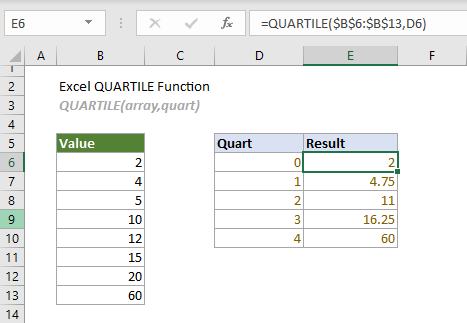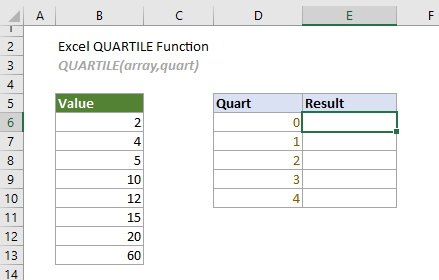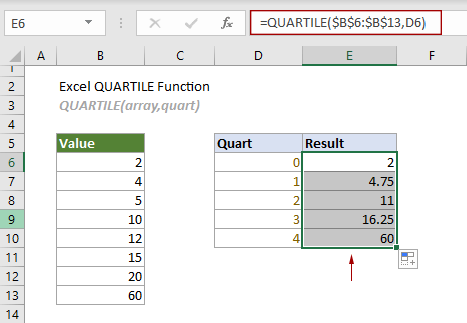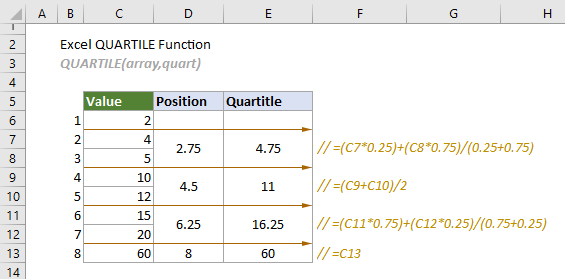## Excel QUARTILE function

The QUARTILE function calculates the quartile for a given set of data. It returns the same value as the QUARTILE.INC function.#### QUARTILE function VS. QUARTILE.INC function:

Starting from Excel 2010, the QUARTILE function has been replaced by the QUARTILE.INC function. Although they can both be used to calculate the quartile of a data set, the QUARTILE.INC function is more recommended for future use, because the QUARTILE function may not be available in future versions of Excel.

#### Syntax

QUARTILE(array, quart)

#### Arguments

• Array (required): The array or cell range for which you want the quartile value;
• Quart (required): A number between 0 and 4 indicates which quartile value to return.
 Number Quartile value 0 Minimum value 1 The 1st quartile (25th percentile) 2 The 2nd quartile - median value (50th percentile) 3 The 3rd quartile (75th percentile) 4 The 4th quartile - maximum value (100th percentile)

#### Remarks

1. If “quart” is not an integer, it will be truncated;
2. The #NUM! error occurs when either of the following conditions is met;
-- “array” is empty;
-- “quart” < 0 or “quart” > 4.
3. The #VALUE! error occurs if “quart” is non-numeric;
4.If the requested quartile falls between two of the values in the “array”, Excel will interpolate between these values to calculate the quartile.

#### Return value

It returns a numeric value.

#### Example

Here is a list of values as shown in the screenshot below, to calculate the minimum value, first quartile, second quartile, third quartile and maximum value by using the QUARTILE function, you can do as follows.Select the top cell, E6 in this case, copy or enter the formula below and press Enter to get the result of the minimum value. Select this result cell and drag it AutoFill Handle down to get other quartiles.

=QUARTILE(\$B\$6:\$B\$13,D6)Notes:

1) The formula in cell E6 can be changed to:

=QUARTILE({2,4,5,10,12,15,20,60},0)

2) The formula can be broken into the following two equations:

2.1 Firstly, apply the following equations to find the position of each quartile in the array:

(In the following equations, n is the total number of cells in the array.)

2.1.1 The equation for calculating the position of Q1:
= 1/4*(n-1)+1
In this case, the position of Q1 is 1/4*(8-1)+1 = 2.75, which means that the first quartile is located between the second and third digits of the array.
2.1.2 The equation for calculating the position of Q2:
= 2/4*(n-1)+1
In this case, the position of Q2 is 2/4*(8-1)+1 = 4.5, which means that the second quartile is located between the fourth and fifth digits of the array.
2.1.3 The equation for calculating the position of Q3:
= 3/4*(n-1)+1
In this case, the position of Q3 is 1/4*(8-1)+1 = 6.25, which means that the third quartile is located between the sixth and seventh digits of the array.
2.1.4 The equation for calculating the position of Q4:
= 4/4*(n-1)+1
In this case, the position of Q4 is 1/4*(8-1)+1 = 8, which means that the fourth quartile is located exactly in the eighth cell of the array.

2.2 Then, return the quartiles based on the positions.

2.2.1 As the position of Q1 (2.75) is closer to the third digit, the second digit is weighted 25% and the third digit is weighted 75%. Then you can apply the following formula to return the first quartile:
=(C7*0.25)+(C8*0.75)/(0.25+0.75) // result is 4.75
2.2.2 Q2 is at position 4.5 of the array, which happens to be the average of the 4th and 5th digits:
=(C9+C10)/2 // result is 11
2.2.3 As the position of Q3 (6.25) is closer to the sixth digit, the sixth digit is weighted 75% and the seventh digit is weighted 25%. Then you can apply the following formula to return the first quartile:
=(C11*0.75)+(C12*0.25)/(0.75+0.25) // result is 16.25
2.2.4 Q4 is exactly at the eighth position of the array, so it will be equal to the value of the cell in this position:
=C13 // result is 60
See the following screenshot:#### Related Functions

Excel QUARTILE.EXC function
The QUARTILE.EXC function returns the quartile for a given set of data based on a percentile range of 0 to 1 exclusive.

Excel QUARTILE.INC function
The QUARTILE.INC function returns the quartile for a given set of data based on a percentile range of 0 to 1 inclusive.

Excel RANK function
The RANK function returns the rank of a number compared to other numbers in the same list.

Excel RANK.AVG function
The RANK.AVG function returns the rank of a number compared to other numbers in the same list.

Excel RANK.EQ function
The RANK.EQ function returns the rank of a number compared to other numbers in the same list.

### The Best Office Productivity Tools

#### Kutools for Excel - Helps You To Stand Out From Crowd

Would you like to complete your daily work quickly and perfectly? Kutools for Excel brings 300 powerful advanced features (Combine workbooks, sum by color, split cell contents, convert date, and so on...) and save 80% time for you.

• Designed for 1500 work scenarios, helps you solve 80% Excel problems.
• Reduce thousands of keyboard and mouse clicks every day, relieve your tired eyes and hands.
• Become an Excel expert in 3 minutes. No longer need to remember any painful formulas and VBA codes.
• 30-day unlimited free trial. 60-day money back guarantee. Free upgrade and support for 2 years.#### Office Tab - Enable Tabbed Reading and Editing in Microsoft Office (include Excel)

• One second to switch between dozens of open documents!
• Reduce hundreds of mouse clicks for you every day, say goodbye to mouse hand.
• Increases your productivity by 50% when viewing and editing multiple documents.
• Brings Efficient Tabs to Office (include Excel), Just Like Chrome, Firefox, And New Internet Explorer.